# Decision Tree Algorithm | Decision Tree in Python | Machine Learning Algorithms | Edureka

4 Oct 2018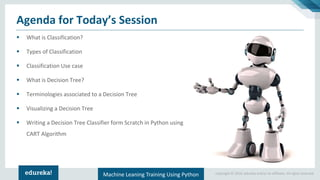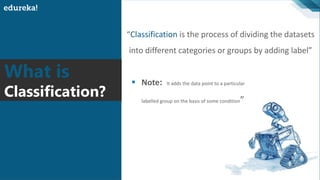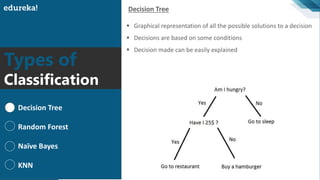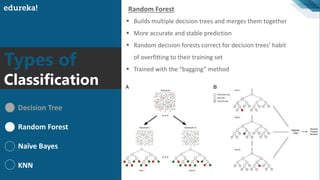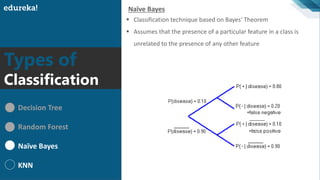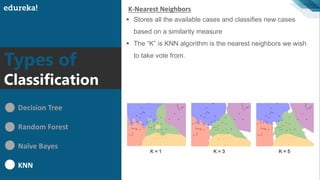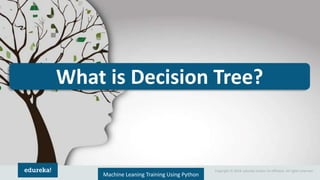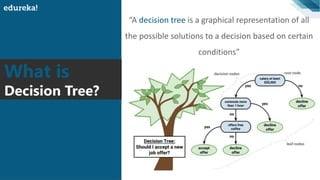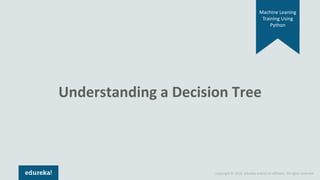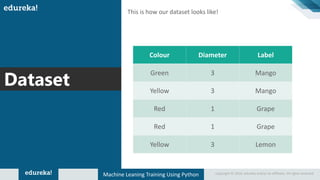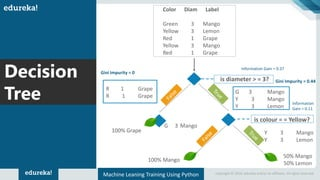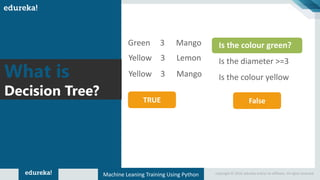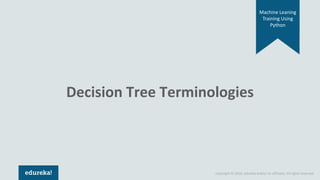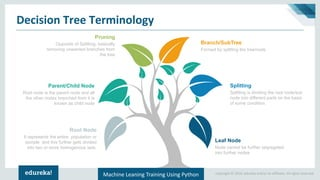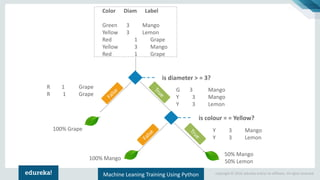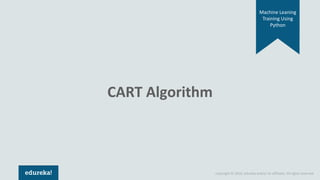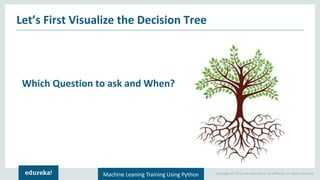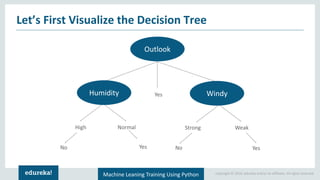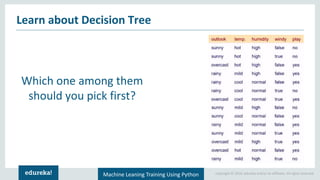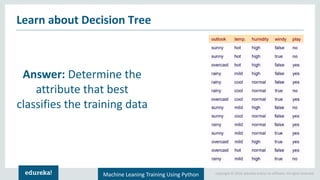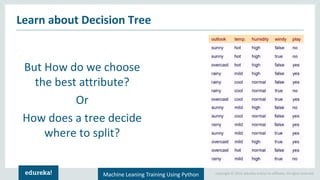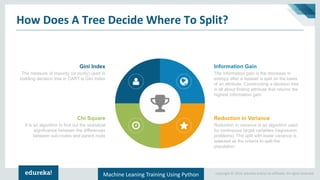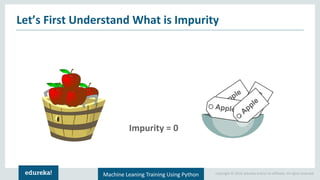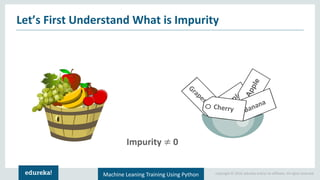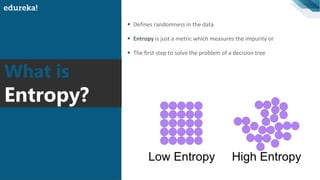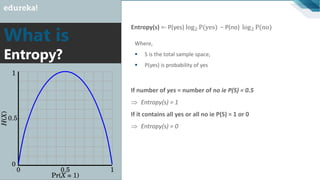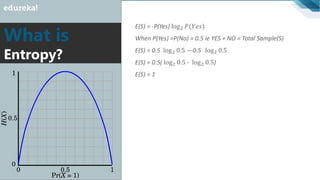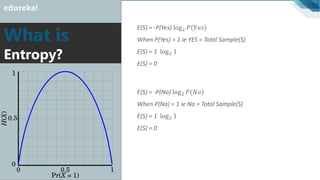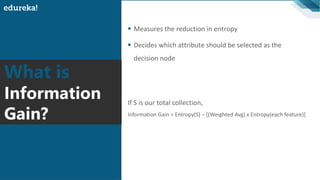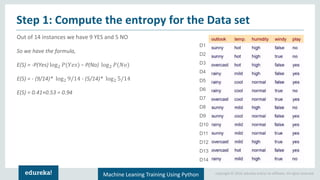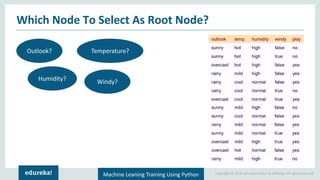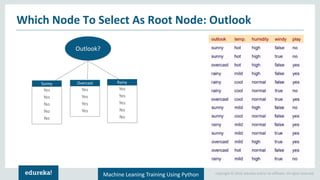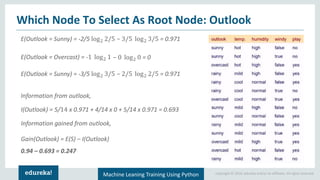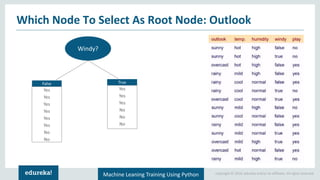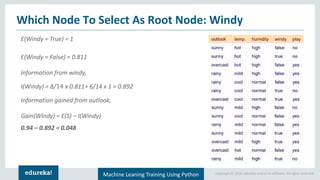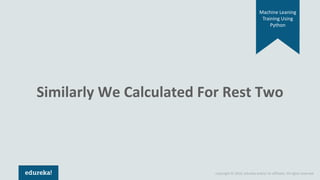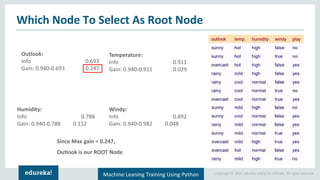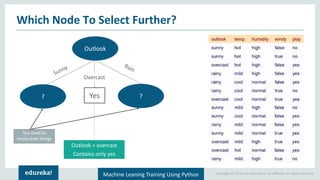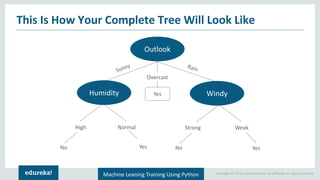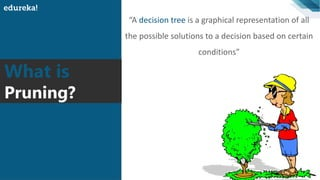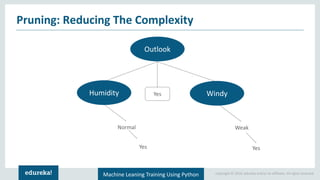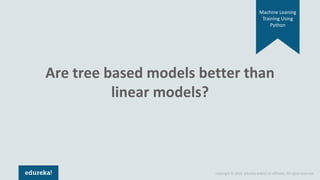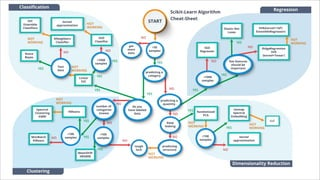1 sur 48

### Decision Tree Algorithm | Decision Tree in Python | Machine Learning Algorithms | Edureka

• 1. Machine Learning Using Python
• 2. Copyright © 2018, edureka and/or its affiliates. All rights reserved.Machine Leaning Training Using Python Agenda for Today’s Session ▪ What is Classification? ▪ Types of Classification ▪ Classification Use case ▪ What is Decision Tree? ▪ Terminologies associated to a Decision Tree ▪ Visualizing a Decision Tree ▪ Writing a Decision Tree Classifier form Scratch in Python using CART Algorithm
• 4. “Classification is the process of dividing the datasets into different categories or groups by adding label” What is Classification? ▪ Note: It adds the data point to a particular labelled group on the basis of some condition”
• 5. Types of Classification Decision Tree Random Forest Naïve Bayes KNN Decision Tree ▪ Graphical representation of all the possible solutions to a decision ▪ Decisions are based on some conditions ▪ Decision made can be easily explained
• 6. Types of Classification Decision Tree Random Forest Naïve Bayes KNN Random Forest ▪ Builds multiple decision trees and merges them together ▪ More accurate and stable prediction ▪ Random decision forests correct for decision trees' habit of overfitting to their training set ▪ Trained with the “bagging” method
• 7. Types of Classification Decision Tree Random Forest Naïve Bayes KNN Naïve Bayes ▪ Classification technique based on Bayes' Theorem ▪ Assumes that the presence of a particular feature in a class is unrelated to the presence of any other feature
• 8. Types of Classification Decision Tree Random Forest Naïve Bayes KNN K-Nearest Neighbors ▪ Stores all the available cases and classifies new cases based on a similarity measure ▪ The “K” is KNN algorithm is the nearest neighbors we wish to take vote from.
• 10. “A decision tree is a graphical representation of all the possible solutions to a decision based on certain conditions” What is Decision Tree?
• 12. Copyright © 2018, edureka and/or its affiliates. All rights reserved.Machine Leaning Training Using Python Colour Diameter Label Green 3 Mango Yellow 3 Mango Red 1 Grape Red 1 Grape Yellow 3 Lemon Dataset This is how our dataset looks like!
• 13. Copyright © 2018, edureka and/or its affiliates. All rights reserved.Machine Leaning Training Using Python ` Decision Tree is diameter > = 3? Color Diam Label Green 3 Mango Yellow 3 Lemon Red 1 Grape Yellow 3 Mango Red 1 Grape G 3 Mango Y 3 Mango Y 3 Lemon R 1 Grape R 1 Grape is colour = = Yellow? Y 3 Mango Y 3 Lemon G 3 Mango Gini Impurity = 0.44 Gini Impurity = 0 Information Gain = 0.37 Information Gain = 0.11 100% Grape 100% Mango 50% Mango 50% Lemon
• 14. Copyright © 2018, edureka and/or its affiliates. All rights reserved.Machine Leaning Training Using Python Is the colour green? Is the diameter >=3 Is the colour yellow TRUE False Green 3 Mango Yellow 3 Lemon Yellow 3 Mango ` What is Decision Tree?
• 16. Copyright © 2018, edureka and/or its affiliates. All rights reserved.Machine Leaning Training Using Python Decision Tree Terminology Pruning Opposite of Splitting, basically removing unwanted branches from the tree Root Node It represents the entire population or sample and this further gets divided into two or more homogenous sets. Parent/Child Node Root node is the parent node and all the other nodes branched from it is known as child node Splitting Splitting is dividing the root node/sub node into different parts on the basis of some condition. Leaf Node Node cannot be further segregated into further nodes Branch/SubTree Formed by splitting the tree/node
• 17. Copyright © 2018, edureka and/or its affiliates. All rights reserved.Machine Leaning Training Using Python is diameter > = 3? Color Diam Label Green 3 Mango Yellow 3 Lemon Red 1 Grape Yellow 3 Mango Red 1 Grape is colour = = Yellow? G 3 Mango Y 3 Mango Y 3 Lemon R 1 Grape R 1 Grape 100% Grape Y 3 Mango Y 3 Lemon 100% Mango 50% Mango 50% Lemon
• 20. Copyright © 2018, edureka and/or its affiliates. All rights reserved.Machine Leaning Training Using Python Let’s First Visualize the Decision Tree No Yes NormalHigh Yes WeakStrong No Yes Outlook WindyHumidity
• 23. Copyright © 2018, edureka and/or its affiliates. All rights reserved.Machine Leaning Training Using Python Learn about Decision Tree But How do we choose the best attribute? Or How does a tree decide where to split?
• 24. Copyright © 2018, edureka and/or its affiliates. All rights reserved.Machine Leaning Training Using Python How Does A Tree Decide Where To Split? Information Gain The information gain is the decrease in entropy after a dataset is split on the basis of an attribute. Constructing a decision tree is all about finding attribute that returns the highest information gain Gini Index The measure of impurity (or purity) used in building decision tree in CART is Gini Index Reduction in Variance Reduction in variance is an algorithm used for continuous target variables (regression problems). The split with lower variance is selected as the criteria to split the population Chi Square It is an algorithm to find out the statistical significance between the differences between sub-nodes and parent node
• 27. What is Entropy? ▪ Defines randomness in the data ▪ Entropy is just a metric which measures the impurity or ▪ The first step to solve the problem of a decision tree
• 28. What is Entropy? If number of yes = number of no ie P(S) = 0.5  Entropy(s) = 1 If it contains all yes or all no ie P(S) = 1 or 0  Entropy(s) = 0 - P(yes) log2 P(yes) − P(no) log2 P(no)Entropy(s) = Where, ▪ S is the total sample space, ▪ P(yes) is probability of yes
• 29. What is Entropy? E(S) = -P(Yes) log2 𝑃(𝑌𝑒𝑠) When P(Yes) =P(No) = 0.5 ie YES + NO = Total Sample(S) E(S) = 0.5 log2 0.5 − 0.5 log2 0.5 E(S) = 0.5( log2 0.5 - log2 0.5) E(S) = 1
• 30. What is Entropy? E(S) = -P(Yes) log2 𝑃(𝑌𝑒𝑠) When P(Yes) = 1 ie YES = Total Sample(S) E(S) = 1 log2 1 E(S) = 0 E(S) = -P(No) log2 𝑃(𝑁𝑜) When P(No) = 1 ie No = Total Sample(S) E(S) = 1 log2 1 E(S) = 0
• 31. What is Information Gain? ▪ Measures the reduction in entropy ▪ Decides which attribute should be selected as the decision node If S is our total collection, Information Gain = Entropy(S) – [(Weighted Avg) x Entropy(each feature)]
• 33. Copyright © 2018, edureka and/or its affiliates. All rights reserved.Machine Leaning Training Using Python Out of 14 instances we have 9 YES and 5 NO So we have the formula, E(S) = -P(Yes) log2 𝑃(𝑌𝑒𝑠) − P(No) log2 𝑃(𝑁𝑜) E(S) = - (9/14)* log2 9/14 - (5/14)* log2 5/14 E(S) = 0.41+0.53 = 0.94 Step 1: Compute the entropy for the Data set D1 D2 D3 D4 D5 D6 D7 D8 D9 D10 D11 D12 D13 D14